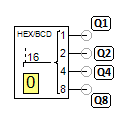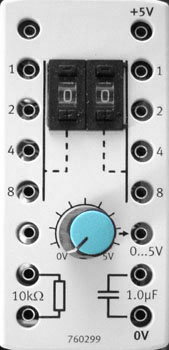# Hex switchWith the hex switch, a number x from 0 to 15 can be output bit-by-bit via the four outputs Q1, Q2, Q4 and Q8. The number x corresponds to a single-digit hexadecimal number, hence the name hex switch. The following applies for the number x:

x = Q1*2^0 + Q2*2^1 + Q4*2^2 + Q8*2^3